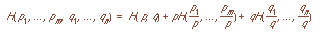# Outline for April 2, 1997

1. Greetings and Felicitations
1. Please speak into the microphone
2. What is cryptography?
1. cipher v. code
2. plaintext (cleartext) M, ciphertext C, key k
3. encryption Ek, decryption Dk
3. Requirements for a cryptosystem
1. enciphering, deciphering is efficient for all keys
2. easy to use
3. strength is depends on secrecy of keys only, not on secrecy of E or D
4. What it can do: Secrecy
1. computationally infeasible to determine Dk from C even if corresponding M known
2. computationally infeasible to determine M from C if k unknown
5. What it can do: Data Authenticity (Integrity)
1. computationally infeasible to determine Ek from C even if corresponding M known
2. computationally infeasible to find a C' such that Dk(C') is valid plaintext
6. Attacks
1. ciphertext only
2. known plaintext
3. chosen plaintext
4. chosen ciphertext
7. Measures of strength
1. unconditionally secure (theoretically unbreakable)
2. conditionally secure (unbreakable in practise); note environment often affects this
3. weak (breakable)
8. How to measure strength: Entropy
1. Given C, how much uncertainty in M?
2. attacker wants 0
3. sender wants lg(n), where n is the length of message
9. Requirements for function H(p1, ..., pn); explain these with dice, coins, and (maybe) horses
1. Review probability notation; assume p1 + ... + pn = 1.
2. H(p1, ..., pn) is a maximum when p1 = ... = pn = 1/n.
3. For any permutation ¼ (Greek letter pi) on (1, 2, ..., n), H(p1, ..., pn) = H(p¼(1), ..., p¼(n)). In other words, H must be a symmetric function of the arguments p1, ..., pn.
4. H is continuous in its arguments
5. H(p1, ..., pn) >= 0 and equals 0 only when one of the pi = 1.
6. H(p1, ..., pn, 0) = H(p1, ..., pn).
7.8. If m and n are positive integers, then9. Let p = p1 + ... + pm and q = q1 + ... + qn, where each pi and qj are non-negative; then, if p and q are positive, with p + q = 1, we must have10. Only function which does this is10. Examples
1. rate for message of length n is r = H(X)/n; for English, 1 to 1.5
2. absolute rate: assume all chars are equally likely; assuming L chars, R = lg L; for English, 4.7
3. redundency D = R - r; for English, 3.2 to 3.7

You can get this document in Postscript, ASCII text, or Framemaker version 5.1.
Notes by Ricardo Anguiano: [LaTeX] [Postscript] [Text]Send email to cs253@csif.cs.ucdavis.edu.

Department of Computer Science
University of California at Davis
Davis, CA 95616-8562